## Notes on Einstein Field Equation

Metric tensor: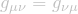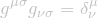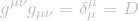(D: dimension of the spacetime)

Christoffel symbol: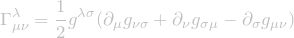Riemann tensor:Ricci tensor:Ricci scalar (curvature scalar):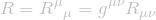Trace of energy-momentum tensor:Einstein field equation:or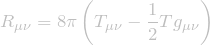Torsion tensor (have nothing to do with energy-momentum tensor):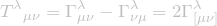Properties of the Riemann tensor (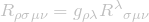):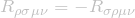(antisymmetric in first two indices)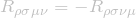(antisymmetric in last two indices)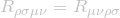(invariant under interchange of the first and last pair of indices)or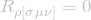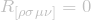Properties of the Ricci tensor: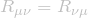Relation between R and T: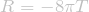Einstein tensor: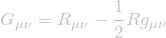so Einstein field equation can be rewritten as: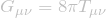Geodesic equation:Covariant derivative: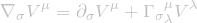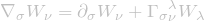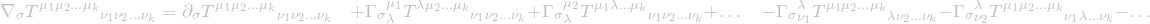Energy-momentum tensor: